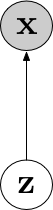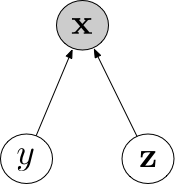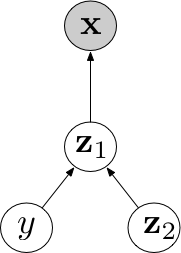Semi-supervised Learning with Deep Generative Models@NIPS15.

## Notations

We are faced with data that appear as pairs $$( \mathbf { X } ,\mathbf { Y } ) = \left\{ \left( \mathbf { x } _ { 1} ,y _ { 1} \right) ,\ldots ,\left( \mathbf { x } _ { N } ,y _ { N } \right) \right\}$$.

We refer to the empirical distribution over the labelled and unlabelled subsets as $$\tilde { p } _ { l } ( \mathbf { x } ,y ) \text{ and } \tilde { p } _ { u } ( \mathbf { x } )$$.

## Latent feature discriminative model (M1)

We construct a deep generative model of the data that is able to provide a more roboust set of latent features:

$p ( \mathbf { z } ) = \mathcal { N } ( \mathbf { z } \vert \mathbf { 0} ,\mathbf { I } ) ; \quad p _ { \theta } ( \mathbf { x } \vert \mathbf { z } ) = f ( \mathbf { x } ; \mathbf { z } ,\theta )$Figure M1

## Generative semi-supervised model (M2)

In addition to a continuous latent variable $$\mathbf { z }$$ some data has class labels $$y$$, we have the following specification of generative process:

$p ( y ) = \operatorname{cat} ( y \vert \pi ) ; \quad p ( z ) = \mathcal { N } ( \mathbf { z } \vert 0,\mathbf { I } ) ; \quad p _ { \theta } ( \mathbf { x } \vert y ,\mathbf { z } ) = f ( \mathbf { x } ; y ,\mathbf { z } ,\theta )$Figure M2

$$y$$ are partly observed.

## M1+M2Figure M1+M2

## Variational distribution

We need to specify the variational distribution $$q_\phi (\mathbf{z}, y \vert x)$$. We assume it has a factorized form:

$q _ { \phi } ( \mathbf { z } ,y \vert \mathbf { x } ) = q _ { \phi } ( \mathbf { z } \vert y, \mathbf { x } ) q _ { \phi } ( y \vert \mathbf { x } )$

Under such a assumption we actually have specified a particular conditional dependency structure of latent variables in the variational distribution. The encoding process can be described as:

$\begin{array}{ll} \text{Sample } y &\sim& q _ { \phi } ( y \vert \mathbf { x } ) \\ \text{Sample } \mathbf{z} &\sim& q_ { \phi } ( \mathbf{z} \vert y, \mathbf { x } ) \end{array}$

When $$y$$ is observed, we only need to sample $$\mathbf{z}$$ from $$q_ { \phi } ( \mathbf{z} \vert y, \mathbf { x } )$$ otherwise we first need to sample $$y$$ from $$q _ { \phi } ( y \vert \mathbf { x } ).$$ In the paper, $$q _ { \phi } ( y \vert \mathbf { x } )$$ is parameterized as Categorical distribution which is hard to reparameterize thus no sampling operation actually happens in the connection between $$q _ { \phi } ( y \vert \mathbf { x } )$$ and $$q_ { \phi } ( \mathbf{z} \vert y, \mathbf { x } )$$, instead what they to actually is to integrate out $$y$$. But if we replace Categorical distribution with its continous approximation Concrete distribution which allows reparameterization trick, then we can unify the operations of $$y$$ and $$\mathbf{z}$$.

In the paper Learning Disentangled Representations with Semi-Supervised Deep Generative Models the authors also relax the assumption about the factorized form of $$q _ { \phi } ( \mathbf { z } ,y \vert \mathbf { x } )$$ which allows more general conditional dependency structure, but the paper seems to be error in equation 4.

## Evidence Lower Bound

Bearing in mind that we have the factorization form of $$q _ { \phi } ( \mathbf { z } ,y \vert \mathbf { x } ) = q _ { \phi } ( \mathbf { z } \vert y, \mathbf { x } ) q _ { \phi } ( y \vert \mathbf { x } )$$ the evidence lower bound can be derived easily.

Labelled data

\left.\begin{aligned} \log p _ { \theta } ( \mathbf { x } ,y ) & \geq \mathbb { E } _ { q _ { \phi } } ( \mathbf { z } \vert \mathbf { x } ,y ) \left[ \log p _ { \theta } ( \mathbf { x } \vert y ,\mathbf { z } ) + \log p ( y ) + \log p ( \mathbf { z } ) - \log q _ { \phi } ( \mathbf { z } \vert \mathbf { x } ,y ) \right] \\ &=: -\mathcal{L}(\mathbf{x}, y) \end{aligned} \right.

Unlabelled data

\left.\begin{aligned} \log p _ { \theta } ( \mathbf { x } ) & \geq \mathbb { E } _ { q _ { \phi } } ( \mathbf { z } ,y \vert \mathbf { x } ) \left[ \log p _ { \theta } ( \mathbf { x } \vert y ,\mathbf { z } ) + \log p ( y ) - \log p ( \mathbf { z } ) - \log q _ { \phi } ( \mathbf { z } ,y \vert \mathbf { x } ) \right] \\ & = : - \mathcal { U } ( \mathbf { x } ) \end{aligned} \right.

Replacing the latent variables posterior joint distribution with its factorization form we get

$\mathcal { U } ( \mathbf { x } ) = \mathcal { H } \left[ q _ { \phi } ( y \vert \mathbf { x } ) \right] - \sum _ { y } q _ { \phi } ( y \vert \mathbf { x } ) \mathcal { L } ( \mathbf { x }, y )$

Combining the labelled and unlabelled loss together:

$\mathcal { J } = \sum _ { ( \mathbf { x } ,y ) \sim \tilde { p } _ { l } } \mathcal { L } ( \mathbf { x } ,y ) + \sum _ { x \sim \tilde { p } _ { u } } \mathcal { U } ( \mathbf { x } )$

The distribution $$q_\phi ( y \vert \mathbf{x} )$$ acts as a predictive distribution and it only contributes to the unlabelled data at present. To remedy this we add it for the labelled data to get

$\mathcal { J } ^ { \alpha } = \mathcal { J } + \alpha \cdot \mathbb { E } _ { \tilde { p } _l ( \mathbf { x } ,y )} \left[ - \log q _ { \phi } ( y \vert \mathbf { x } ) \right]$

We can also consider $$q_\phi ( y \vert \mathbf{x} )$$ as a discriminative classifier; hence the first term is the generative loss whereas the second term is the discriminative loss.